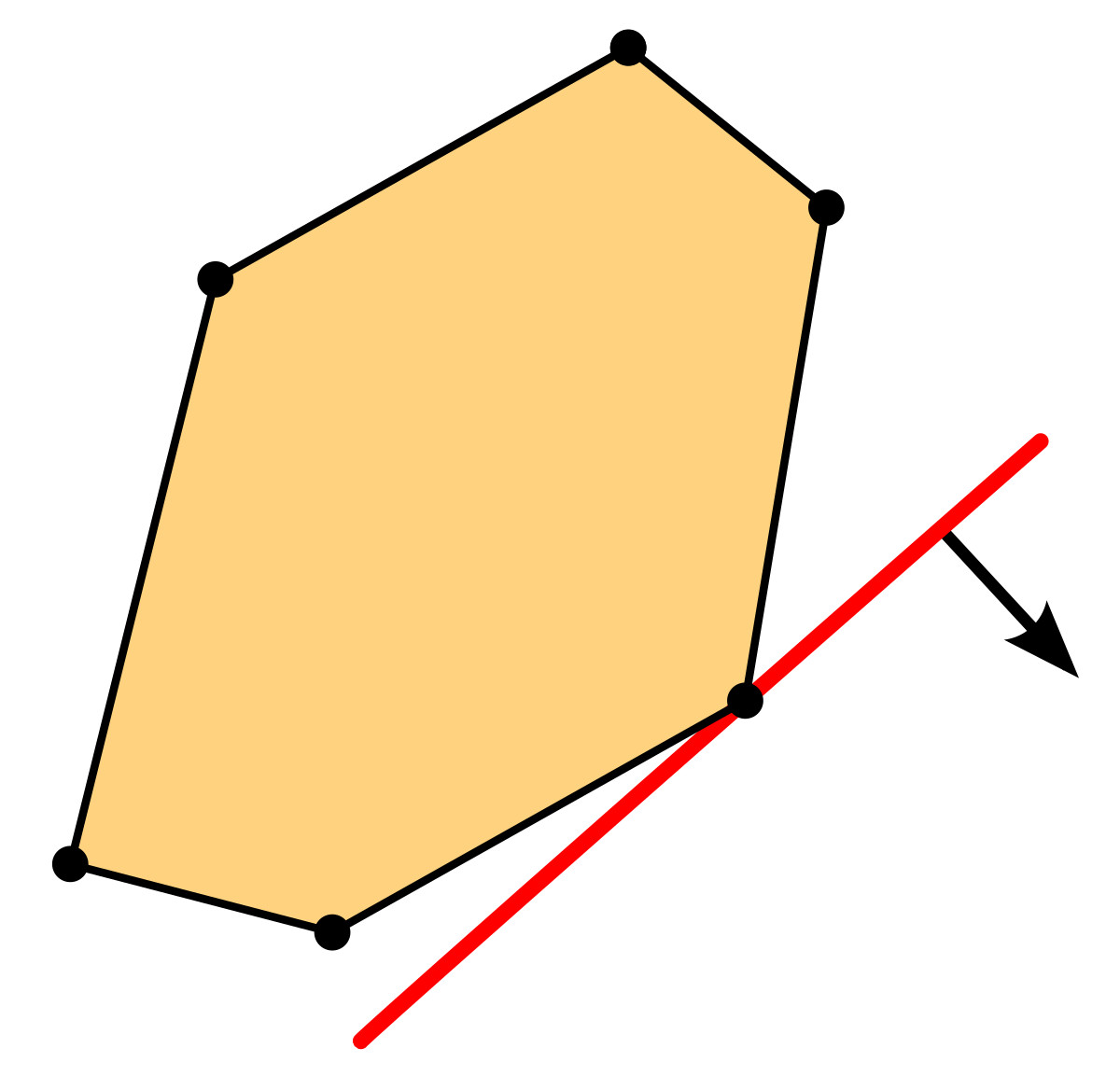### Probability and Stochastic processes

Title : Probability and stochastic processes

Summary of the course :

The aim of this course is to introduce some important classes of stochastic processes, both in discrete and continuous time, which are relevant in Biology, Operation Research, Physics and Social Sciences.

In the first part of the course, we will recall some basic notions in measure theory and their role in the foundations of modern probability theory. Then, after introducing random variables, expectations and conditional expectations, we will cover the basic theory of stochastic processes and their applications. The main topics here will be random walks and discrete and continuous time Markov chains. The course will also cover some important applications in mathematical biology and, if time permits, in mathematical finance.

Prerequisites :

Basic probability, linear algebra and measure theory.

Keywords :

Random variables, conditional expectations, stochastic processes, Markov chains in discrete and continuous time, applications in mathematical biology and in mathematical finance.

### Linear and Semidefinite Optimization

Summary of the course :

The course covers the basic aspects of linear and semidefinite optimization. We address the duality theory, highlighting the differences of LP and SDP,  the simplex method for LP, complementarity and optimality conditions, interior-point methods. Examples from applications in data science, decision theory and financial mathematics will be analysed, such as portfolio optimization, scheduling, transportation problems, network design. Practical experiments will be performed with Matlab/Scilab.

Prerequisites :

Basic linear algebra and convex geometry.

Keywords :

Conic programming, duality, optimality conditions, simplex method, interior-point method, software experiments.### UE M1MA-01 - Numerical analysis of PDE

The aim of this coourse is to provide you a significant introduction to numerical analysis of PDE and computation by using FreeFem++.